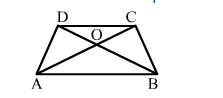# Assertion: In a trapezium ABCD we have AB || DC and the diagonals AC and BD intersect at O. Then, ar(∆AOD) = ar(∆BOC)

Question:

Assertion: In a trapezium ABCD we have AB || DC and the diagonals AC and BD intersect at O. Then, ar(AOD) = ar(BOC)Reason: Triangles on the same base and between the same parallels are equal in areas.
(a) Both Assertion and Reason are true and Reason is a correct explanation of Assertion.
(b) Both Assertion and Reason are true but Reason is not a correct explanation of Assertion.
(c) Assertion is true and Reason is false.
(d) Assertion is false and Reason is true.

Solution:

(a) Both Assertion and Reason are true and Reason is a correct explanation of Assertion.

In trapezium ABCD, ∆ABC and ABD  are on the same base and between the same parallel lines.
∴ ar(∆ABC) = ar(∆ABD)
⇒ ar(∆ABC-">- ar(∆AOB) = ar(∆ABD) -">- ar(∆AOB)
⇒ ​ar(BOC) = ar(AOD
∴ Assertion (A) is true and, clearly, reason (R) gives (A).